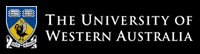School of Computer Science and Software Engineering

# School of Computer Science and Software Engineering

## CITS4406 Problem Solving and Programming

#### Labsheet 6: Loops and Decision Structures

This labsheet is in two parts: a number of review exercises that reinforce concepts from the lectures; and an important problem-solving technique, motivated by its use in solving a larger-sized problem representative of the types of problems that can be solved with computers.

#### Review Exercises:

1. Write a function that reads the marks.txt file and prints the names of the student who obtained maximum marks in each subject. e.g. "Tom Hanks got the highest marks of 90 in CITS1401".
2. Write a function that will calcuate the average marks obtained in each subject in the above file and print them along with the subject name.
3. If passing marks in each subject are 50, write a function that will print the number of students failed in each subject.
4. Write a function that will calculate and print the overall total marks of each student along with the student's name.
5. Forensic document examination is the process of examining questioned documents e.g. to detect forgery. Generally, handwritten documents are examined but in this new age, typed documents (using a computer) are becoming more common. Thus, it is sometimes required to identify the author of an anonymous document from the writing style. This is called linguistic stylometry. You can also read about it on Wikipedia. I has legal, academic and literacy applications including authorship identification of historic, anonymous and disputed documents.

Write a Python function that reads a text file and generates a writing style "signature" of the writer. The signature should basically encode the frequecy of some common words that appear in every document. For example the frequency of the word "for" is the number of times "for" appears divided by the total number of words in the ducument.

By using common words such as 'the', 'for', 'and', 'is', 'are', and even full stops and punctuations, it is possible to match the signature of an anonymous document to documents with known authorships even when the contents of the documents are completely different.

Your task is to generate stylometric signatures (list of float values) of the following e-books.

halfHearted.txt
huntingtower.txt
roughingIt.txt
lifeOnMississippi.txt

More e-books are available here.
6. Write a Python function that will compute the Euclidean distance between two stylometric signatures (lists of float elements) computed in the above question. Refer to this link for equation of Euclidean distance.
7. Plot the signatures using graphics.py and different colours for visual inspection of the signature patterns.

#### Problem Solving Technique: Backtracking

Consider the "verbal arithmetic" puzzle SEND + MORE = MONEY. The requirement is to assign one of the digits 0, 1, ..., 9 consistently to each of the eight letters in the puzzle so that the arithmetic works correctly. There are various constructive ways to solve this type of puzzle which depend on logic and maths, but what is a good generic approach?

One way is to use enumeration, as in Lab 5. We might assign a digit to D, then assign a different digit to E, then assign different ones to each of the other letters, and when the assignment is complete, check whether the arithmetic works. But of course the problem is that with ten digits and eight letters, there are 1,814,400 distinct assignments, so this would be quite slow, even for such a small puzzle.

A better way is to use backtracking, which is similar to enumeration except that every time we assign a digit to a letter, we check immediately whether a solution is still possible. So if we assign 4 to D, then 7 to E, then 2 to Y, we can say immediately that we have gone wrong, because the sum in the rightmost column doesn't work (4 + 7 = 11, so Y can't be 2). The early termination of this sequence of assignments saves a lot of work and makes the process much faster.

As a second example, consider doing a Sudoku puzzle. If we reach a point where we can't identify any square with only one possible number, then we might guess a number for one of the squares, then continue the puzzle under the assumption that the guess was correct. Then if at some later time we hit an impossible situation, we go back - we backtrack - to that guess and try a different number. This also is backtracking.

The basic structure of backtracking is as follows:

def bt(soln):
if not reject(soln):
if accept(soln):
print(soln)
else:
for each way of extending soln:
bt(extension of soln)

and we invoke this function with bt(initial soln).

To apply backtracking to a real problem, we need to provide four facilities:

• the initial (usually empty) solution;
• a way of rejecting a partial solution;
• a way of accepting a complete solution;
• and a way of identifying all ways of extending a solution.
For verbal arithmetic:
• the initial solution would be the empty assignment;
• a partial solution can be rejected if the arithmetic doesn't work, even with some values unknown;
• a complete solution can be accepted if the arithmetic works;
• and extending a solution would mean assigning a free digit to a free letter.

PROBLEM SOLVING QUESTION 6.1) The classic problem solved using backtracking is the 8-queens problem: place eight queens on a standard chess board such that no queen attacks any other queen. (Recall that a queen can move any number of squares horizontally, vertically, or diagonally, so effectively we are asking that no queens share a row, column, or diagonal.) The more-general version is the n-queens problem, which extends the idea to chess boards of size n x n.

Define the four facilities outlined above and put them into the backtracking framework to construct a Python funcion nqueens(n) that generates all solutions to the n-queens problem, and displays them using turtle graphics.

# The University of Western Australia

## University information

CRICOS Code: 00126G